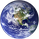TradingView1311瀏覽
Co-variance is a representation of the average percent data points deviate from there mean. A standard calculation of Co-variance uses One standard Deviation. Using the empirical rule, we can assume that about 68.26% of Data points lie in this range.

The advantage to plotting co variance as a time series is that it will show you how volatility of a trailing period changes. Therefore trend lines and other methods of analysis such as Fibonacci retracements could be applied in order to generate volatility targets.

For the purpose of this indicator I have the mean using a vwma derived from vwap . This makes this measurement of co-variance more sensitive to changes in volume , likewise are more representative a change in volatility , thus giving this indicator a "leading aspect".

//Moving Covariance by Rashad
study(title="Moving Covariance", shorttitle="MCV", overlay=false)
src = vwap, len = input(30, minval=1, title="Length")
mean = vwma(src, len)
stdev = stdev(src, len)
covariance = (stdev/mean)*100
plot(covariance, title = "moving covairance", style=line, linewidth = 2, color = red)


## 評論I would like to apologize, when naming this indicator I mixed up my terminology. This is coefficent of variation which shows the % a price deviates from its mean. Also known as unitized risk.